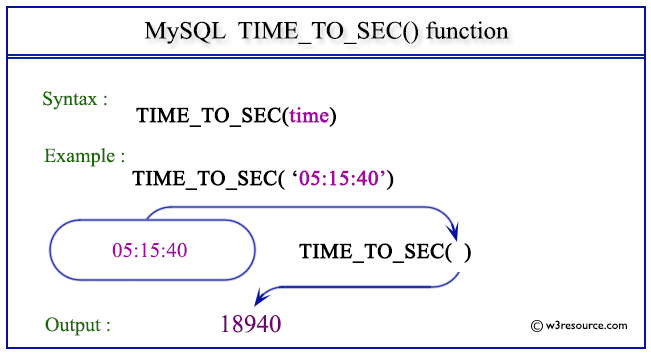# MySQL TIME_TO_SEC() function

## TIME_TO_SEC() function

MySQL TIME_TO_SEC() converts a time value in to seconds that have elapsed since midnight. It is a valuable tool for time-based calculations and comparisons.

This function is useful in -

• TIME_TO_SEC() is valuable for comparing time values based on their numeric representation, facilitating time-related comparisons.
• It can be used to calculate the duration between two time points by subtracting the converted seconds.
• TIME_TO_SEC() supports time analysis by providing a common unit of measurement, enabling time-related statistics and trends to be calculated.
• It is useful for filtering records or data based on time criteria, such as selecting records within a specific time range.
• The function is used in generating time-based reports with time values presented in seconds, which can be useful for data analysis.
• The function aids in sorting time values in ascending or descending order based on their numeric representation.
• It supports time series analysis by providing a consistent representation of time values for modeling and forecasting.

Syntax:

```TIME_TO_SEC(tm)
```

Where tm is a time.

Syntax Diagram:MySQL Version: 8.0

Pictorial Presentation:Example:

The following statement will convert the specified time 05:15:40 in seconds.

Code:

``````SELECT TIME_TO_SEC('05:15:40');
```
```

Output:

```mysql> SELECT TIME_TO_SEC('05:15:40');
+-------------------------+
| TIME_TO_SEC('05:15:40') |
+-------------------------+
|                   18940 |
+-------------------------+
1 row in set (0.02 sec)
```

Video Presentation:

All Date and Time Functions: